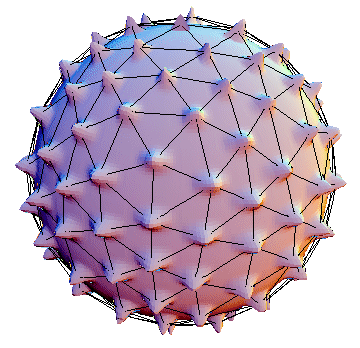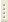RUS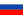Theoretical department of ISSP RAS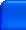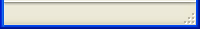Collective excitations in multicomponent quasi-two-dimensional electron systems The study of collective excitations in multicomponent quasi-two-dimensional electron systems, such as quantum wells with several levels of dimensional quantisation, quasi-two-dimensional electron systems with several filled Landau levels in strong magnetic field, double tunnel coupled quantum wells is one of the main research directions of the theoretic department. (i) The theory of intersubband charge density and spin density excitations for electrons in quantum well in strong magnetic field is developed. It is shown that the excitations have a multimode structure, the number of modes being determined by filling factor. (V.E.Bisti. Pis'ma v ZhETF, 69, 543, 1999; V.E.Bisti, Pis'ma v ZhETF, 73, 25, 2001). (ii) Collective intrasubband charge density excitations are investigated in double GaAs/AlGaAs quantum wells in external parallel magnetic field. It is shown that in strong parallel magnetic fields the anisotropy of plasmon energy is determined by electron effective mass anisotropy due to finite width of the quantum well. (S.V. Tovstonog, V.E. Bisti. Pis'ma v ZhETF, 78, 1237, 2003).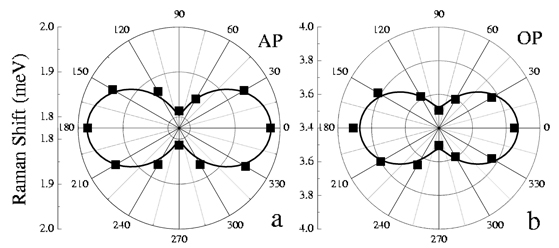Dependence of the energy (Raman shift) for AP (left) и OP (right) in the 250/20/250 Å double quantum well on the angle between the excitation momentum (k=13·104 cm-1) and magnetic field direction (B||=7 T) in polar coordinates. Black squares are experimental data, line presents the calculated results. Goldstone mode relaxation in a quantum Hall ferromagnet Spin relaxation in strongly correlated 2DEG placed in strong magnetic field is studied for filling factors close to odd numbers. The considered system is a Quantum Hall Ferromagnet possessing "classical" spin S0~Nj/2 (Nj being the number of magnetic flux quanta, S0||B). The spin relaxation time is actually the time of Zeeman energy relaxation (rather than spin dephasing time) due to the processes changing the 2DEG spin. The SO-interaction Rashba and Dresselhaus terms are considered as factors mixing the spin states. The relaxation is studied in terms of 2D magnon annihilation processes. The problem is solved for arbitrary values of the initial perturbation by employing the analytical Exciton Representation technique. The temporal behaviour of S(t) was found to be non-exponential (S.Dickmann. Goldstone Mode Relaxation in a Quantum Hall Ferromagnet. Phys. Rev. Lett., 93, 206804 (2004); S.M.Dickmann, V.M.Zhilin, D.M.Kulakovski. Excitonic representation. Spectra of collective excitations in the Quantum Hall effect regime. Spin biexciton. ZhETF, 128, issue 5(11), 1025-1040 (2005)).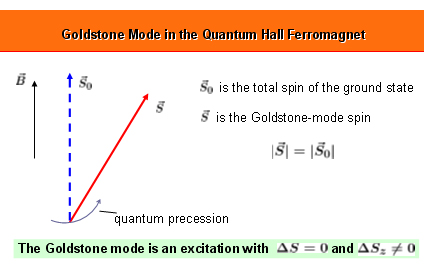The total spin can be tilted as awhole from the direction of B (DS=0, DSz≠0), i.e. the initial perturbation can be described as a spontaneous symmetry breaking. From the microscopic point of view, this mode decomposition due to the presence of a smooth random potential(at T < 1 K) occurs through the disapperance of the "Goldstone" Bose-condensate of "zero" 2D magnons with a simultaneous development of the "thermodynamic" condensate.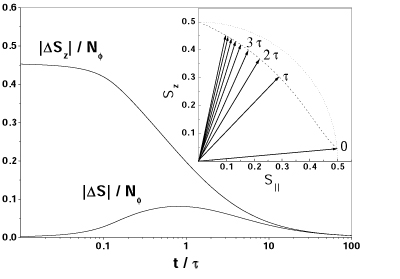The Goldstone mode relaxation. Time dependences of |DSz| and |DS| are shown for the initial perturbation |DSz(0)|=0.455Nj. The vectors S(t) at equidistant moments are plotted in the inset with step t. Characteristic time of the Goldstone mode relaxation should be weakly dependent on the magnetic field: t ≈ t0 exp(g B3/2), where t0 ~ 10 ns and g ~ 0.01 T -3/2. Edge magnetoplasmons in 2D systems with smoothly vanishing mobile charge carriers density at the boundary Two-dimensional systems with the density of mobile charge carriers smoothly vanishing at the boundary placed in normal magnetic field were predicted to possess anomalous edge magnetoplasmons whose frequency at fixed wavevector along the boundary changes non-monotonously with magnetic field rising from 0 at H=0 to a peak value at some magnetic field and then gradually falling again to zero at large H. These anomalous magnetoplasmons were later disovered experimentally in the two-dimensional system of positive He ions localized at the liquid helium surface (S.S.Nazin and V.B.Shikin. On edge magnetoplasoms in the electron system on the surface of liquid helium. Long wavelength asymptotics of the spectrum. (Sov. Phys. JETP, 67 (1988) 288).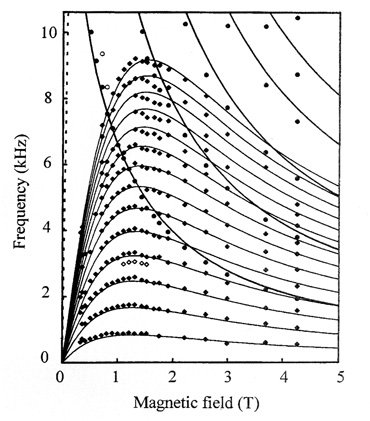Low-frequency plasma oscillation spectrum in the two-dimensional disk-like (radius R=11.87 mm) system of positive helium ions at temperature T=55 mK for 2D ion density n=8x106 cm-2. Dots are the experimental results while curves are obtained by numerical calculations. Non-monotonous curves correspond to anomalous edge magnetoplasmons. Monotonously falling curves represent ordinary edge magnetoplasmons (P.L.Elliot et al., Magnetoplasmons in two-dimensional circular sheets of 4He+ ions. Phys. Rev., B56 (1997) 3447--3456).   Quantum Hall effect in non-uniform 2D electron systems Transport measurements in 2D charged systems almost always introduce inhomogineity in the studied sample due to the contact phenomena. However, although it is desirable to have the maximum possible homogeneity for the observation of Quantum Hall Effect, it is clearly observed in such systems. A theory of the Quantum Hall Effect (QHE) in non-uniform 2D charged systems is being developed which provides a natural explanation for this contradiction. Plotted in the figure is an example of the self-consistent description of electrostatic fields in 2D electron disk in the normal state and in the QHE regime.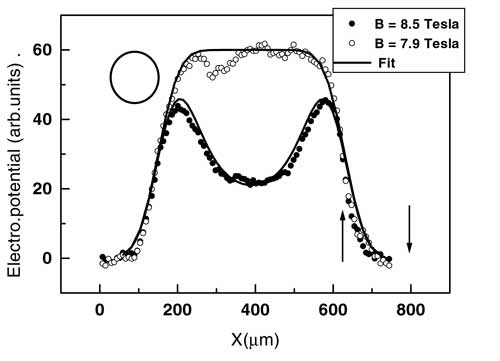Gauss transform f(x) of the electric potential j(x) for the Corbino disk with the 2D domain centered at x0=390 m m. Inset at the right illustrates the magnetic fields corresponding to the considered states; circle at the left gives an idea of the actual size of the laser beam used. The interval between the arrows in the right bottom corner corresponds to the overlap of normal and anomalous states in the bahaviour of potential j(x). Its finite width indicates the existence of edge normal rings around anomalous domain at the center of the disk.   Light-induced Hall effect in conductors with broken mirror symmetry The interest to conductors (semiconductors, normal metals, and superconductors) with broken mirror symmetry of the crystal lattice is due to the expected new physical properties of such materials compared to ordinary crystals possessing the point reflection symmetry. In particular, a new physical property of semiconductors and metals is predicted related to the absence of mirror symmetry. It is shown that irradiation of quasi-two-dimensioanl and three-dimensional conductors possessing polar symmetry by external alternating circularly polarized electric field results in deviation of the direction of direct current proportional to the intensity of incident radiation. The effect is similar to the Hall effect, the difference being that the role of external magnetic field is played by circularly polarized light. (V.M.Edelstein. Phys. Rev. Lett., 95, 156602 (2005)).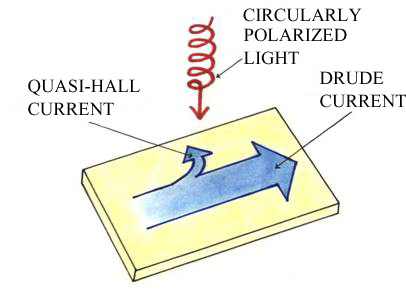Meso- and nanostructures Various meso- and nanostructures, such as nanotubes, fullerenes, clusters, and bubbles (both charged and neutral) in condensed matter are the objects of permanent interest. Shown in the figure is the predicted at the ISSP RAS multielectron bubble in liquid helium in the state where 2D electron system at its internal boundary forms a Wigner lattice.